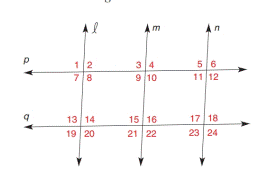Chapter 2.3, Problem 7E### Elementary Geometry for College St...

6th Edition
Daniel C. Alexander + 1 other
ISBN: 9781285195698

#### Solutions

Chapter
Section### Elementary Geometry for College St...

6th Edition
Daniel C. Alexander + 1 other
ISBN: 9781285195698
Textbook Problem
1 views

# In Exercise 7 to 16, name the lines (if any) that must be parallel under the given conditions.Exercise 7-16 ∠ 1 ≅ ∠ 20

To determine

To find:

The parallel lines.

Explanation

Given:

p and q are cut by transversal .

120

Approach:

(1) If two lines are cut by a transversal so that two corresponding angles are congruent, then these lines are parallel.

(2) Vertically opposite angles are congruent to each other.

Calculation:

The given statement is,

p and q are cut by transversal .

120

1 and 8 are vertically opposite angles

### Still sussing out bartleby?

Check out a sample textbook solution.

See a sample solution

#### The Solution to Your Study Problems

Bartleby provides explanations to thousands of textbook problems written by our experts, many with advanced degrees!

Get Started

#### In Problems 7-34, perform the indicated operations and simplify. 22.

Mathematical Applications for the Management, Life, and Social Sciences

#### Determine whether f'(0) exists. f(x)={xsin1xifx00ifx=0

Single Variable Calculus: Early Transcendentals, Volume I

#### True or False: Du f(a, b) = ∇(a, b) · u.

Study Guide for Stewart's Multivariable Calculus, 8th